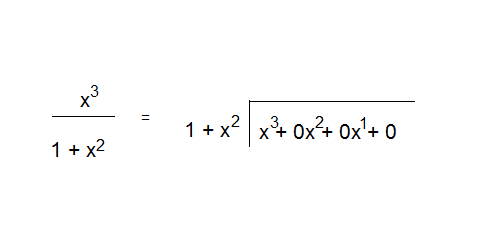# Algebraic Manipulation within Integration by Parts problem

Lebombo

## Homework Statement

I'm doing an integration by parts problem. After setting up [∫udv = uv - ∫vdu], I come across this situation. How is this algebraic manipulation accomplished?

From: $\int\frac{x^{3}}{1+x^{2}}dx$

To: $\int x - \frac{x}{1+x^{2}}dx$

Homework Helper
Gold Member
Dearly Missed
By polynomial long division, for example.

Lebombo
I dread polynomial long division because I never had the opportunity to learn it in school. Skipped most of college algebra due to military and never took precalc, which has created a lot of struggling spots in Calculus

How do I even set this up for long division? I gave it a try in the attached picture. Is this how it should be set up? Or should I rewrite the numerator portion from [1+x^2] to [x^2 + x^1 + 1] as done with the denominator?Last edited:
brmath
Strangely, it is exactly the same as elementary school long division.

Set your divisor up as ##x^2 + 1## instead of ## 1 + x^2##. Then divide ##x^2 \text{ into }x^3## getting a (partial) quotient of x. Place that on top of your division sign just as you did in 5th grade long division.

Continue with your 5th grade long division by multiplying your x by ##x^2 + 1## and placing the product below the ##x^2 + 1##. Now subtract as you would in an ordinary problem. In this case what you have is a remainder, of -x so you put that over the divisor ##x^2 + 1##.

While you do at this point have two integrals that are easy to compute, the step was not necessary. You could have done the original by substitution: u = ##x^2##. Try it.

Staff Emeritus
Homework Helper
What you have started is fine. If you learned how to do long division with numbers, division with polynomials is quite similar.

Step 1: what factor must you multiply (1+x^2) by to obtain an expression with x^3 in it?

Step 2: write the factor from step 1 on top over the x^3

Step 3: multiply the factor from Step 1 by (1 + x^2) and write the result below the polynomial inside the bracket. Write like powers underneath like powers.

Step 4: Subtract the product from the original polynomial being divided.

Step 5: If the result of the subtraction is a polynomial of smaller degree than(1+x^2), you can stop. Otherwise, repeat steps 1-4 above.

The quotient will be the entire expression on top of the brackets, and the remainder will be the expression of smaller degree than the divisor which is at the bottom of all of the subtractions.

Enjoy!